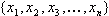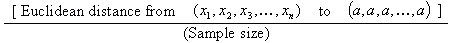From: Sonia, a university teaching assistant I'm a teaching assistant for an undergraduate level statistics class. One student asked today why we don't just take the average of the absolute value of difference scores (use the mean deviation) to describe variability instead of calculating the standard deviation. I wasn't completely sure how to answer her question. What is the actual answer? I remember asking myself the same thing. Just like the standard deviation, the mean deviation is a measure of how much a sample deviates from being identically equal to the average. It is better in that its meaning is clear: it is the "average farness" from an individual to the average. (I have never been told if there is anything "standard"' about the standard deviation). You can represent the two parameters geometrically on small samples: On a samplewith average a, the standard deviation is:For instance, on a sample of 2: {x,y} with average a = (x+y)/2, the standard deviation is half of the euclidian distance from (x,y) to (a,a) and the mean deviation is half of the rectilinear distance from (x,y) to (a,a); and on a sample of 3: {x,y,z} with average a = (x+y+z)/3, the standard deviation is one third of the euclidian distance from (x,y,z) to (a,a,a) and the mean deviation is one third of the rectilinear distance from (x,y,z) to (a,a,a). The euclidian distance is a more natural parameter than the rectilinear distance, and that is how I have come to understand why the standard deviation appears more naturally in computations than the mean deviation. Claude